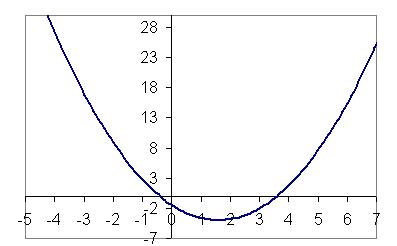#### You may also likeA circle is inscribed in an equilateral triangle. Smaller circles touch it and the sides of the triangle, the process continuing indefinitely. What is the sum of the areas of all the circles?### Areas and Ratios

Do you have enough information to work out the area of the shaded quadrilateral?### Climbing Powers

$2\wedge 3\wedge 4$ could be $(2^3)^4$ or $2^{(3^4)}$. Does it make any difference? For both definitions, which is bigger: $r\wedge r\wedge r\wedge r\dots$ where the powers of $r$ go on for ever, or $(r^r)^r$, where $r$ is $\sqrt{2}$?

# Root Hunter

##### Age 16 to 18Challenge Level

Take a look at the function in the graph below.The graph is positive for $x = 5$ and negative for $x = 3$. This means that the graph must cut the $x$ axis somewhere between $3$ and $5$.
Although in this case the result is obvious (because we have the whole graph to look at!), we can also use this idea to show that more tricky functions also have roots.

Use this idea to show that these functions possess at least one solution $f(x) = 0$:

$$f(x)=\frac{1}{x-2}+\frac{1}{x-3}$$ $$f(x)= x^x - 1.5 x$$ $$f(x)= x^{1000000}+{1000000}^x - 17$$ $$f(x)=\cos(\sin(\cos x)) - \sin(\cos(\sin x))$$

Optional extension activity: Can you make a spreadsheet that helps you find the numerical values of the roots to, say, four decimal places?